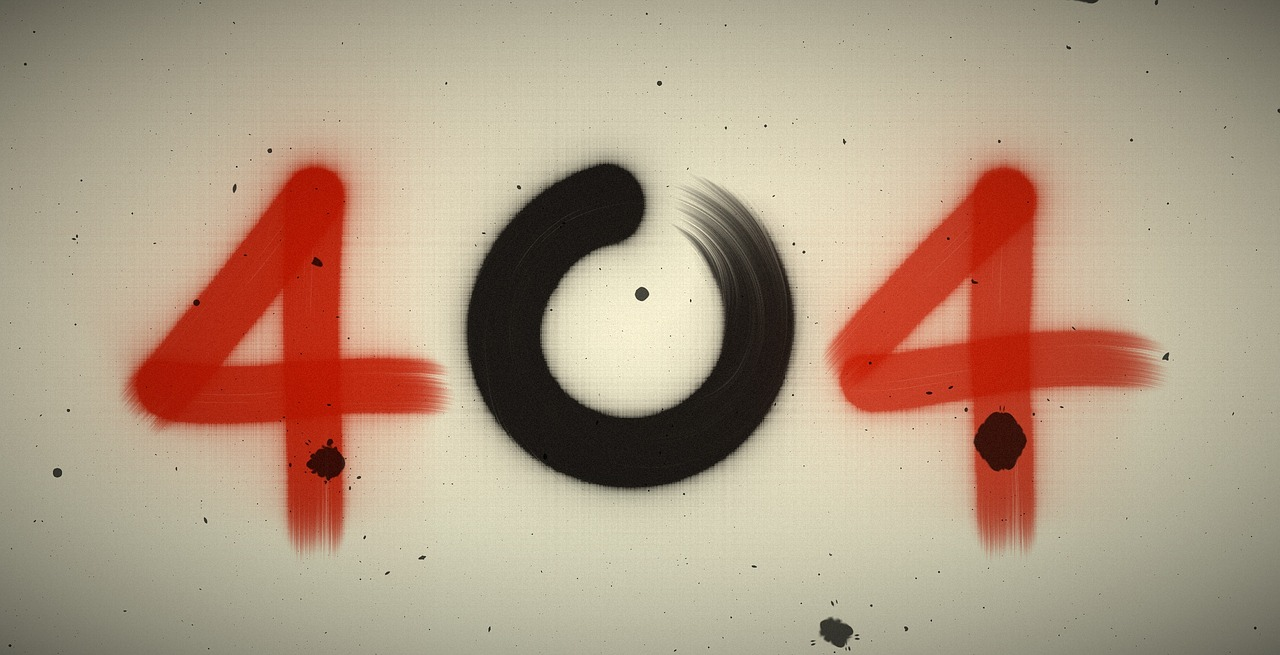Our page on Facebook with over 2 million followers was deleted. Follow the new page here: Physics-Astronomy.com Facebook Page

## Post per Page

Back in May 2016, the world's lengthiest math proof was assembled. The complete proof requires nearly 200 terabytes, beating out the earlier record-holding proof, which took "only" 13 gigabytes. The Boolean Pythagorean Triples problem was initially postured in the 1980s by a California-based mathematician named Ronald Graham. The Boolean Pythagorean Triples problem, which was cracked and verified by Marijn J. H. Heule, Oliver Kullmann, and Victor W. Marek, revolves around the Pythagorean theorem: a^2 + b^2 = c^2. There are precise sets of positive integers, known as Pythagorean triples, which can be added into the formula, for instance 32 + 42 = 52, and 52 + 122 = 132.

Now suppose that every positive integer is one of red or blue. Graham enquired if it's imaginable to color all the integers either red or blue so that there will be no set of Pythagorean triples with all the same color. The answer in the record-setting proof states that this is impossible. Learn more about this in the video below: